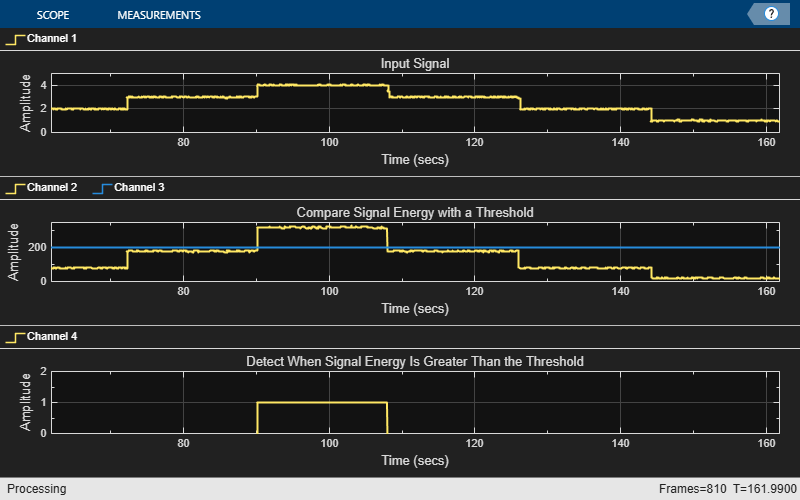## Energy Detection in the Time Domain

This example shows how to detect the energy of a discrete-time signal over a finite interval using the RMS value of the signal. By definition, the RMS value over a finite interval -NnN is given by:

`$RMS=\sqrt{\frac{1}{2N+1}\sum _{n=-N}^{N}|x\left(n\right){|}^{2}}$`

The energy of a discrete-time signal over a finite interval -NnN is given by:

`${E}_{N}=\sum _{n=-N}^{N}|x\left(n\right){|}^{2}$`

To determine the signal energy from the RMS value, square the RMS value and multiply the result by the number of samples that are used to compute the RMS value.

`${E}_{N}=RM{S}^{2}×\left(2N+1\right)$`

To compute the RMS value in MATLAB® and Simulink®, use the moving RMS System object™ and block, respectively.

### Detect Signal Energy

This example shows how to compute the energy of a signal from the signal's RMS value and compares the energy value with a specified threshold. Detect the event when the signal energy is above the threshold.

Create a `dsp.MovingRMS` System object™ to compute the moving RMS of the signal. Set this object to use the sliding window method with a window length of 20. Create a `timescope` object to view the output.

```FrameLength = 20; Fs = 100; movrmsWin = dsp.MovingRMS(20); scope = timescope('SampleRate',Fs,... 'TimeSpanOverrunAction','Scroll',... 'TimeSpanSource','Property','TimeSpan',100,... 'ShowGrid',true,'LayoutDimensions',[3 1],'NumInputPorts',3); ```
```scope.ActiveDisplay = 1; scope.YLimits = [0 5]; scope.Title = 'Input Signal'; ```
```scope.ActiveDisplay = 2; scope.YLimits = [0 350]; scope.Title = 'Compare Signal Energy with a Threshold'; ```
```scope.ActiveDisplay = 3; scope.YLimits = [0 2]; scope.PlotType = 'Stairs'; scope.Title = 'Detect When Signal Energy Is Greater Than the Threshold'; ```

Create the input signal. The signal is a noisy staircase with a frame length of 20. The threshold value is 200. Compute the energy of the signal by squaring the RMS value and multiplying the result with the window length. Compare the signal energy with the threshold value. Detect the event, and when the signal energy crosses the threshold, mark it as 1.

```count = 1; Vect = [1/8 1/2 1 2 3 4 3 2 1]; threshold = 200; for index = 1:length(Vect) V = Vect(index); for i = 1:90 x = V + 0.1 * randn(FrameLength,1); y1 = movrmsWin(x); y1ener = (y1(end)^2)*20; event = (y1ener>threshold); scope(y1,[y1ener.*ones(FrameLength,1),threshold.*ones(FrameLength,1)],event.*ones(FrameLength,1)); end end ```You can customize the energy mask into a pattern that varies by more than a scalar threshold. You can also record the time for which the signal energy stays above or below the threshold.

Watch now